# How to Convert Horizontal List to Vertical List in Excel?

When we try to perform operations on a horizontal list in Excel, we may encounter some issues, and you may need to convert the same list to a vertical list to make it clearer. Attempting to complete this task manually can be time-consuming. So, we can make the process faster by following some tricks. The tricks we can use are: the first trick is by using the formulas, and the other trick is by using the paste special option. In this tutorial, we will learn how to convert a horizontal list to a vertical list in Excel.

## Convert Horizontal List to Vertical List Using Formulas

Here we will get any one of the values using the INDEX formula, then use the auto-fill handle to get all the results. Let us look at a simple procedure for converting a horizontal list to a vertical list in Excel using formulas.

### Step 1

Consider an Excel sheet with data that is similar to the data shown in the image below.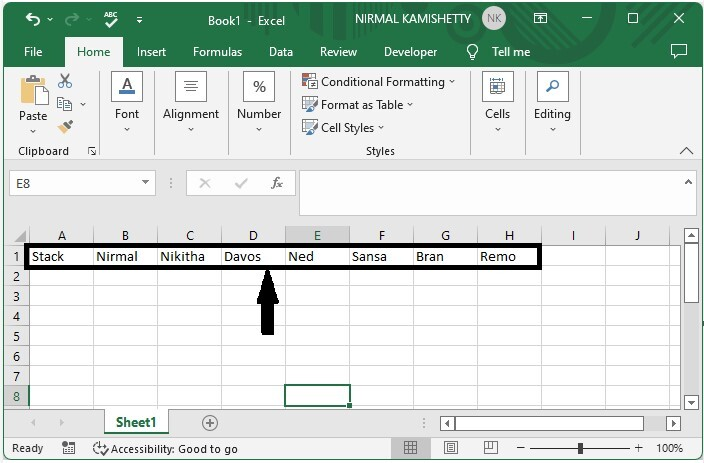Now click on the empty cell in our case, cell J1, and enter the formula as

=INDEX(A$1:L$1, ROWS(M\$1:M1)) and click Enter to get our first value.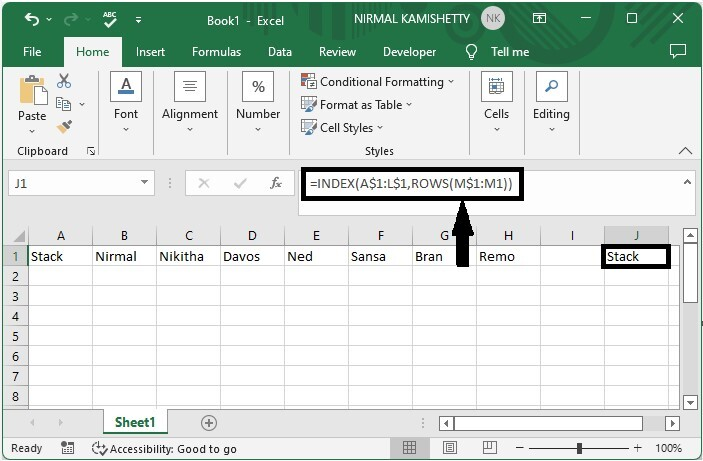### Step 2

Now to get all the values of the list, drag down from the first result using the auto-fill handle, and our vertical list will be similar to the below image.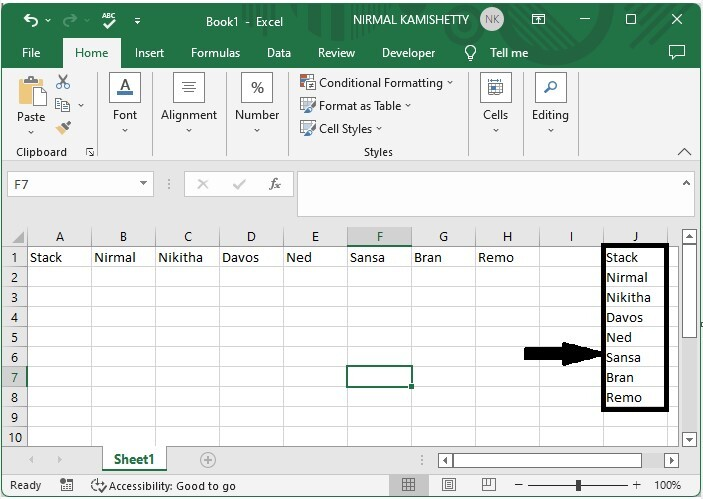## Convert Horizontal List to Vertical List Using Paste Special

Here we will copy the data in the default way and then use the paste special to complete the task. Let us look at a simple procedure for converting a horizontal list to a vertical list in Excel using the paste special.

### Step 1

Consider the same data as in the preceding example.

Now select the list, then use the command CTRL + C to copy the list, then click on the cell you want to start the list from, then click on paste under home and select paste special.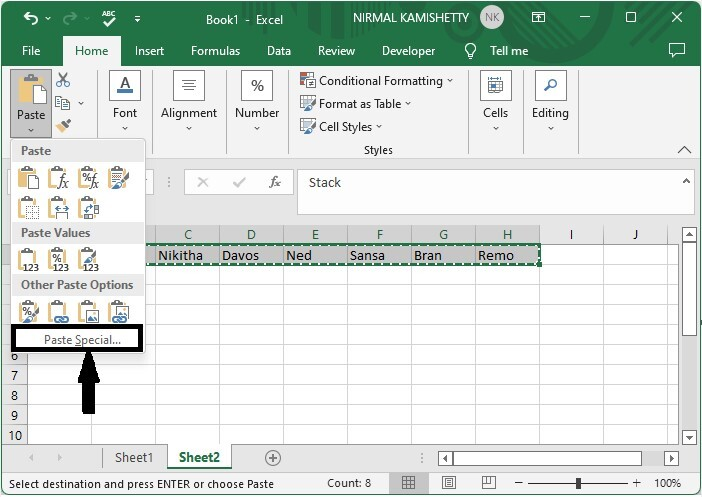### Step 2

Then in the pop-up, tick the box beside "transpose" and click OK to complete our process.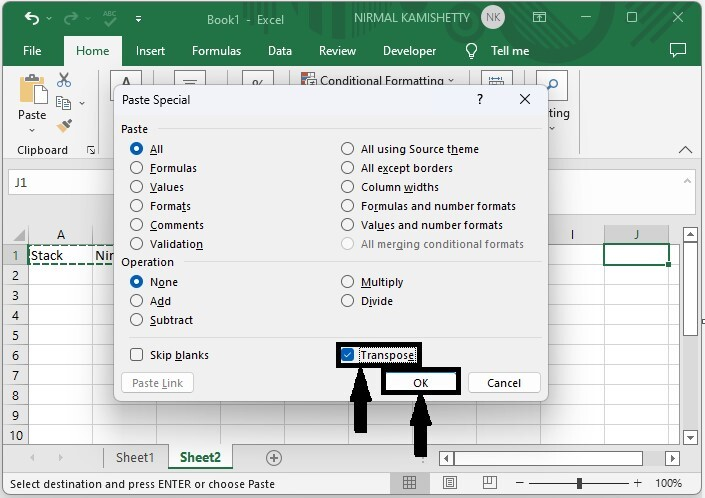## Conclusion

In this tutorial, we use a simple example to demonstrate how you can convert a horizontal list to a vertical list in Excel.

Updated on: 06-Mar-2023

372 Views